# heatmaps

## Flexible plotting of Functional Genomics data + Sequence features

This package provides simple functions for plotting heatmaps over sets of genomic windows.

This vignette is an example workflow using ChIP-seq data in zebrafish: the User Guide contains detailed information on the package internals if you want fine-grained control of your plots or to develop tools which use parts of the package.

First things first, load the `heatmaps` package:

``library(heatmaps)``

`heatmaps` is written using core Bioconductor packages, so reading in data can be easily accomplished with the standard tools.

Here we read in a set of zebrafish promoters from a 30% Epiboly (30p) embryo, defined using CAGE data, and corresponding H3K4me3 ChIP-seq data:

``````library(rtracklayer)
library(GenomicRanges)
library(BSgenome.Drerio.UCSC.danRer7)

heatmaps_file = function(fn) system.file("extdata", fn, package="heatmaps")

zf_30p_promoters = import(heatmaps_file("30pEpi_proms.bed"), genome=seqinfo(Drerio))

h3k4me3_30p = h3k4me3_30p_pos + h3k4me3_30p_neg``````

## Plotting Functional Genomics Data

Many kinds of functional genomics data, such as ChIP-seq, RNA-seq or DNase-seq can be visualised as ‘coverage’ tracks. In UCSC, these would be wig, bigWig or bedGraph files.

First, we need to create our windows. We can create another `GRanges` object which contains 500bp either side of our promoters, using the `promoters` function in `GenomicRanges`. Unfortunately, some of the resulting ranges go off the end of a chromosome and so must be dropped: this is done by testing the width of the trimmed object.

The `CoverageHeatmap` function creates a heatmap object from a `GRanges` object or an `RleList`. If we are using a `GRanges` object then the weight can be specified. Internally, this is passed to the `coverage` function from `GenomicRanges`. In the example we are working with `RleList`s, which are returned by the `coverage` function.

All heatmaps contain a `coords` slot, which lets `plotHeatmap` know how to plot the co-ordinates on the x-axis: very often, our plots will be centered on some feature rather than starting from zero on the x axis. The `label` slot is optional, and is displayed in the top left-hand corner of the plot by default, if present.

``````coords=c(-500, 500)

windows_30p = promoters(zf_30p_promoters, -coords, coords)``````
``````## Warning in valid.GenomicRanges.seqinfo(x, suggest.trim = TRUE): GRanges object contains 1 out-of-bound range located on sequence chr21.
##   Note that ranges located on a sequence whose length is unknown (NA) or
##   on a circular sequence are not considered out-of-bound (use
##   seqlengths() and isCircular() to get the lengths and circularity flags
##   of the underlying sequences). You can use trim() to trim these ranges.
``````windows_30p = windows_30p[width(trim(windows_30p)) == 1000]
h3k4me3_30p_heatmap = CoverageHeatmap(
windows_30p,
h3k4me3_30p,
coords=coords,
label="H3K4me3 30p")``````

The `plotHeatmapList` function will plot the returned heatmap object to the active device. This function also allows multiple plots to be plotted at the same time, and sets the device margins. It is usually easier to `plotHeatmapList` rather than `plotHeatmap` directly.

Options for plotting can be passed to `plotHeatmapList` function. Here, we set the label text size (`cex.label`) to be smaller than the default, and use the default color scheme from `RColorBrewer`. A complete list of color schemes is available using the command `RColorBrewer::display.brewer.all()`, or on the ColorBrewer website.

``plotHeatmapList(h3k4me3_30p_heatmap, cex.label=1, color="Greens")``
``## plotting heatmap H3K4me3 30p``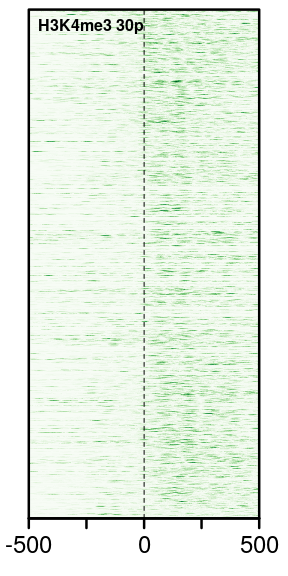Another way of visualising this signal is using a meta-region plot. This is effectively just a sum over the ‘columns’ of a heatmap.

``plotHeatmapMeta(h3k4me3_30p_heatmap)``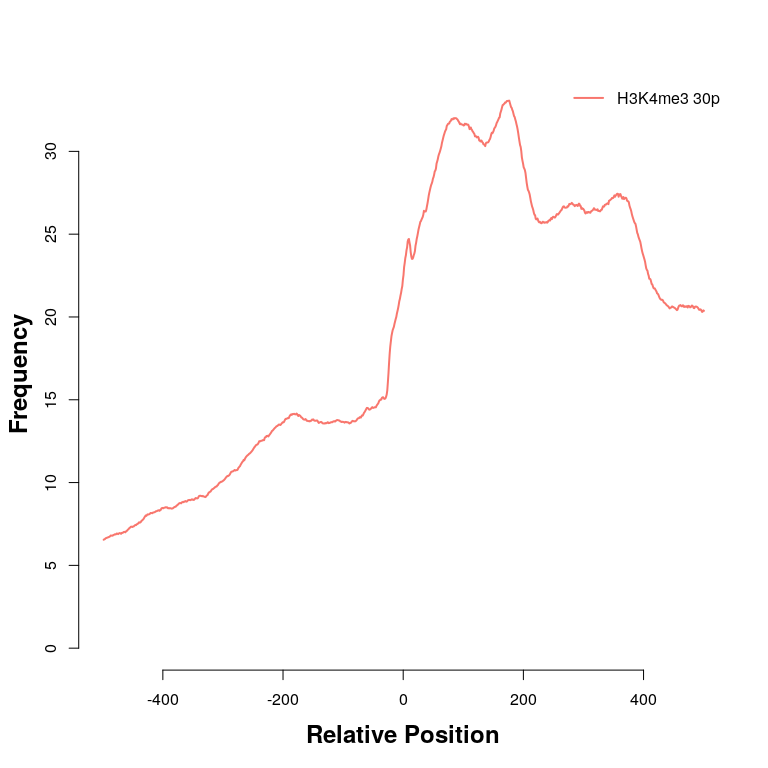We can see from this picture that there is an enrichment of H3K4me3 signal downstream of the promoters. It appears to have some kind of phase, but it’s not very clear what’s happening.

If we subtract negative strand reads from positive strand reads, a better picture starts to emerge.

It is very easy to specify custom color schemes in `heatmaps`. If a vector of colors is supplied (in any format R understands), then they are interpolated by `colorRamp`.

When we are using non-obvious color schemes, it can help to plot a legend describing the value of the colors. This is handled automatically by `plotHeatmapList` if the option `legend=TRUE` is set.

``````h3k4me3_30p_subtracted = h3k4me3_30p_pos - h3k4me3_30p_neg

h3k4me3_30p_subtracted_hm = CoverageHeatmap(
windows_30p,
h3k4me3_30p_subtracted,
coords=coords,
label="Phase")

scale(h3k4me3_30p_subtracted_hm) = c(-150, 150)

plotHeatmapList(h3k4me3_30p_subtracted_hm, cex.label=1.5, color=c("red", "white", "blue"), legend=TRUE, legend.width=0.3)``````
``## plotting heatmap Phase``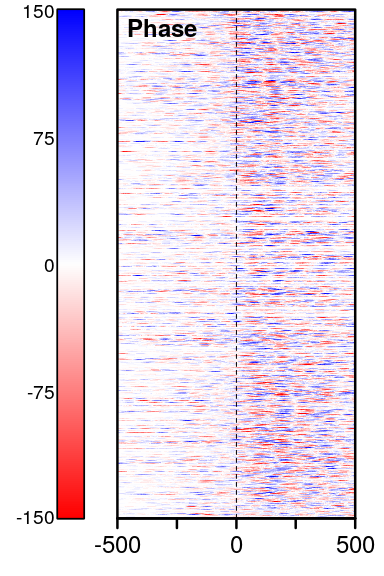It can also be helpful to cluster heatmaps. The `heatmaps` package does not provide methods for clustering, but can display a partition defined by the user. This is to make sure any method can be used, and that the clusters can be recovered after plotting (particularly using non-deterministic methods like k-means).

We can use a simple k-means approach from within R to partition the rows of our image matrix, then re-order the rows, remembering the clustering:

``````raw_matrix = image(h3k4me3_30p_subtracted_hm)
clusters = kmeans(raw_matrix, 2)\$cluster

mat = raw_matrix[order(clusters),]

h3k4me3_30p_subtracted_kmeans = Heatmap(
mat,
coords=coords,
label="kmeans",
scale=c(-150, 150))

plotHeatmapList(h3k4me3_30p_subtracted_kmeans,
cex.label=1.5,
color=c("red", "white", "blue"),
partition=c(sum(clusters==1), sum(clusters==2)),
partition.legend=TRUE,
partition.lines=TRUE,
legend=TRUE,
legend.pos="r",
legend.width=0.3)``````
``## plotting heatmap kmeans``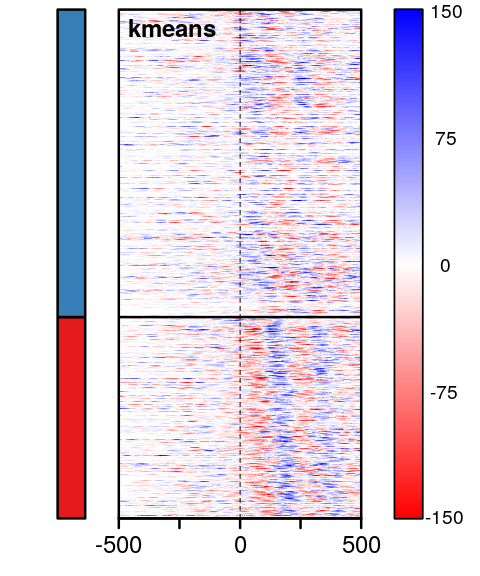## Plotting Sequence Features

`heatmaps` also contains convenient functions to plot sequence features, such as kmer content or PWM matches, or genomic windows.

First we extract the sequence associated with our windows:

``seq_30p = getSeq(Drerio, windows_30p)``

Now we can use the function `PatternHeatmap` to extract patterns from our sequence. We can specify either kmers, including ambiguity codes, or using PWMs.

``````ta_30p = PatternHeatmap(seq_30p, "TA", coords=coords)
plotHeatmapList(ta_30p)``````
``## plotting heatmap TA``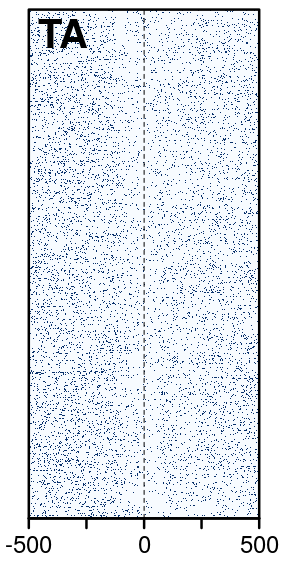This heatmap is difficult to see patterns in because the points are binary and the data is sparse. `heatmaps` provides a function to smooth this data. It also lets us resize the image so that our plots don’t take ages to plot. If we plotted every point individually, the result would be much higher resolution than could possibly fit onscreen. `output.size` specifies the dimensions of the output image matrix.

The `algorithm` argument specifies the smoothing method. Specifying “kernel” uses the `bkde2D` function from the package `KernSmooth`. In this case, because we are using binary data, this would be chosen automatically.

``ta_30p_smoothed = smoothHeatmap(ta_30p, output.size=c(250, 500), algorithm="kernel")``
``````##
## Calculating kernel density...``````
``````## Warning in bkde2D(cbind(df\$i, df\$j), bandwidth = sigma, gridsize =
## output.size, : Binning grid too coarse for current (small) bandwidth: consider
## increasing 'gridsize'``````
``plotHeatmapList(ta_30p_smoothed)``
``## plotting heatmap TA``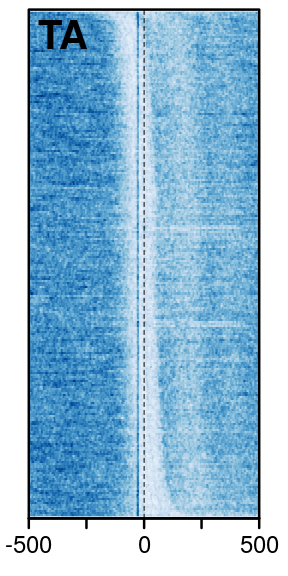Using PWMs instead of kmers is very similar, except we also have to specify a minimum match score. This can either be absolute or expressed as a percentage (see `?Biostrings::matchPWM` for details).

``````example_data = new.env()
data(HeatmapExamples, envir=example_data)
tata_pwm = get("tata_pwm", example_data)

tata_pwm_30p = PatternHeatmap(seq_30p, tata_pwm, coords=coords, label="TATA", min.score="60%")
plotHeatmapList(smoothHeatmap(tata_pwm_30p, output.size=c(250, 500)))``````
``````##
## Calculating kernel density...``````
``## plotting heatmap TATA``